# Problems on Differential band brake

Problems

A differential band brake shown in figure below has an angle of contact of 225°. The band has a lining whose coefficient of frictions is 0.25 and the drum diameter is 400 mm. The brake is to sustain a torque of 375 Nm.

Find (a) the necessary force for the clockwise and counter-clockwise rotation of the drum and

```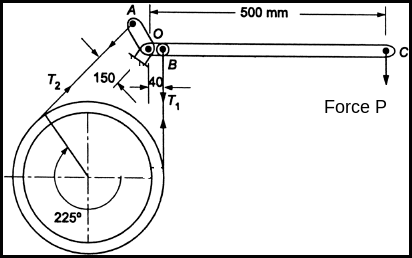Solution:```
```Given data :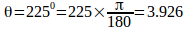,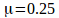,
```
`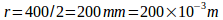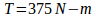`
```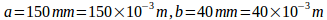```
```First find the tension in
tight side and slack side of the belt,```
`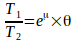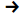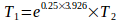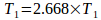`
`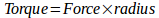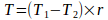`
`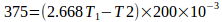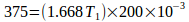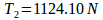`
`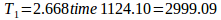`
`a) Force required`
```Clockwise
rotation of the drum ```
`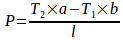`
`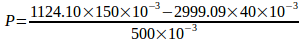`
`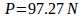`
```
```
`b) Force required`
```Anti-Clockwise
rotation of the drum ```
`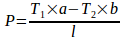`
`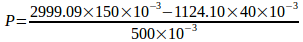`
`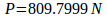`
```
```

2) A differential band brake has a drum radius 400mm. The two ends of the band are fixed to the pins on both sides of fulcrum lever at 40 mm and 200 mm from fulcrum. The angle of contact is 270 degrees. Taking coefficient of friction as 0.2 determine the braking torque developed when a force of 600 N is applied to the lever at a distance of 800 mm from the fulcrum.

```Given data :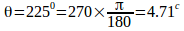,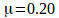,
```
`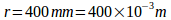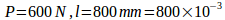`
```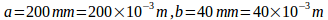```
```First find the tension in
tight side and slack side of the belt,```
`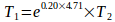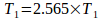`
```{Note: This ratio remain same
for clockwise or anticlockwise rotations }```
```A) For clockwise rotation
of drum```
``
`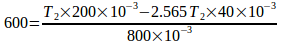`
```
```
`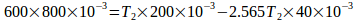`
```
```
`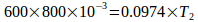`
```
```
`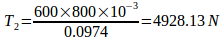`
```
```
```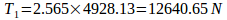```
```Braking torque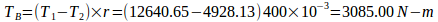```
```A) For Anti-clockwise
rotation of drum```

``
`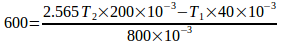`
```
```
`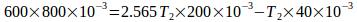`
```
```
`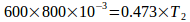`
```
```
`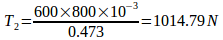`
```
```
```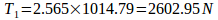```
```Braking torque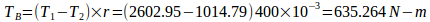```